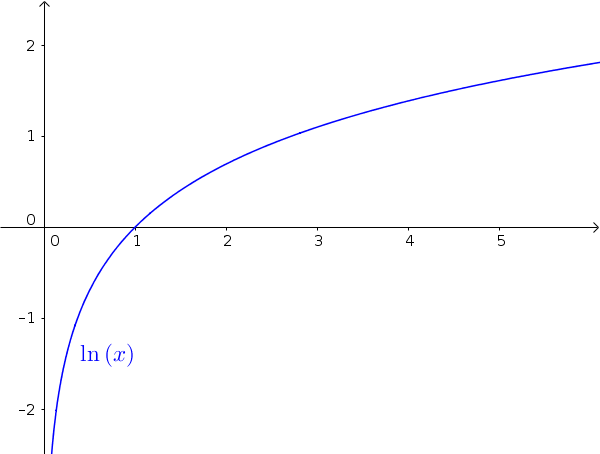Math Natural exponential function Natural logarithm function

# Natural logarithm function

The inverse function of the natural exponential function is the natural logarithm function or ln-function:

$f(x)=\ln(x)$
!

### Remember

The ln-functionis a logarithmic function with the Euler number as a basis:

$\ln(x)=\log_e(x)$i

### Hint

The natural logarithm short $\ln$ is a logarithm with base $e$:
$\ln=\log_e$
!

### Remember

Since the natural logarithm function is the inverse function of the exponential function with base e, the following computation law applies:

$x=\ln(e^x)$ $=e^{\ln(x)}$

Tip: The rule is advantageous in deriving the derivative of general exponential functions.